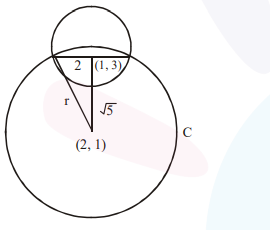# Solve this following

Question:

If one of the diameters of the circle $x^{2}+y^{2}-2 x-6 y+6=0$ is a chord of another circle ' $C$ ', whose center is at $(2,1)$, then its radius is

Solution:$x^{2}+y^{2}+2 x-6 y+6=0$

center $(1,3)$

radius $=2$

distance between $(1,3)$ and $(2,1)$ is $\sqrt{5}$

$\therefore(\sqrt{5})^{2}+(2)^{2}=r^{2}$

$\Rightarrow \mathrm{r}=3$